# EOC Algebra 1 Practice Test #1

EOC Algebra 1 Practice Test #1: Our free EOC Algebra 1 Practice Test is an online quiz designed to help students prepare for the Algebra 1 End-of-Course (EOC) exam. The practice test consists of 40 multiple-choice questions, which are similar in format and content to the questions on the actual Algebra 1 EOC exam.

The practice test covers a variety of algebraic concepts, including linear equations, quadratic equations, functions, systems of equations, exponents, and polynomials. The questions are designed to assess a student’s ability to solve algebraic equations, interpret algebraic expressions, and use algebraic concepts to solve real-world problems.

## EOC Algebra 1 Practice Test #1

The practice test is administered online, allowing students to complete the quiz at their own pace and on their own schedule. Once a student completes the practice test, they will receive a score report that provides feedback on their performance, including the number of questions answered correctly and incorrectly.

Practice Tests
3366

Algebra EOC Practice Test #1

Algebra EOC Practice Test #1
Total Items: 40
Time Limit: 60 minutes
Score: You can find your test score as a percentage at the end of the test!

1 / 40

Divide (16x6 − 12x4 + 4x2) by 4x2

2 / 40

George is helping the manager of the local produce market expand her business by distributing flyers around the neighborhood. He gets paid $20 a day as well as$0.05 for every flyer he distributes. George would like to earn at least $65 each day. Which of the following represents this situation, where x is the number of flyers distributed? 3 / 40 Which graph represents the solutions of p + 1 < −1 OR p − 5 > 7?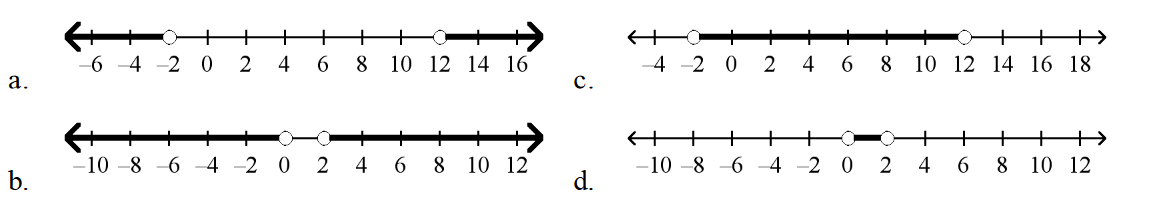4 / 40 John is considering accepting one of two sales positions. ABC Company offers a yearly salary of$45, 000. XYZ Company offers a yearly salary of $38, 000 plus a 2% annual commission on sales. For what amount of sales s is the salary at XYZ Company greater than the salary at ABC Company? 5 / 40 Solve - \frac4s = \frac{-2}{9} 6 / 40 Janell has 5 gallons of paint. After painting 800 square feet of walls in her house, she has 3 gallons left. The graph below show’s Janell’s situation.7 / 40 The scatter plot shows the relationship between the weekly total sales ($) and the number of different rugs designs a rug store has. Based on this relationship, use the line of best fit to predict what the total sales will be when the store has 110 different rug designs.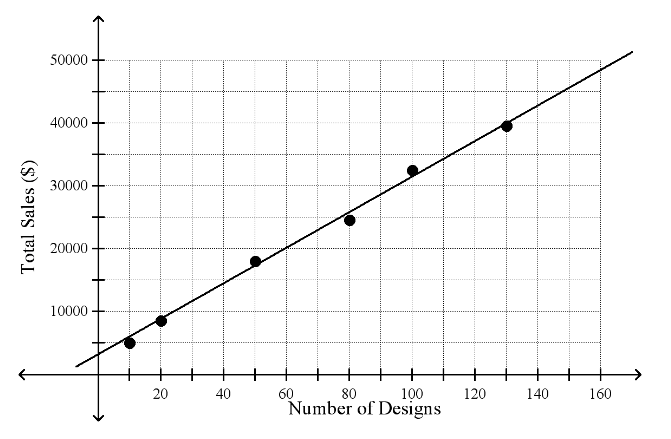8 / 40

Solve x² − 7x − 8 = 0 by factoring.

9 / 40

Give the domain and range of the relation.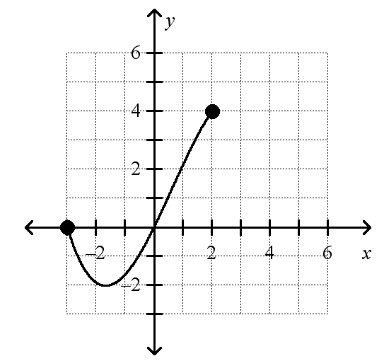10 / 40

Reserved tickets for the football game cost $20 each and general admission tickets cost$12 each. The total ticket sales brought in $900. The equation below can be used to find out how many of each type of ticket were sold, where x is the number of reserved tickets and y is the number of general admission tickets. 20x + 12y = 900 Which of the following graphs shows the graph of this equation?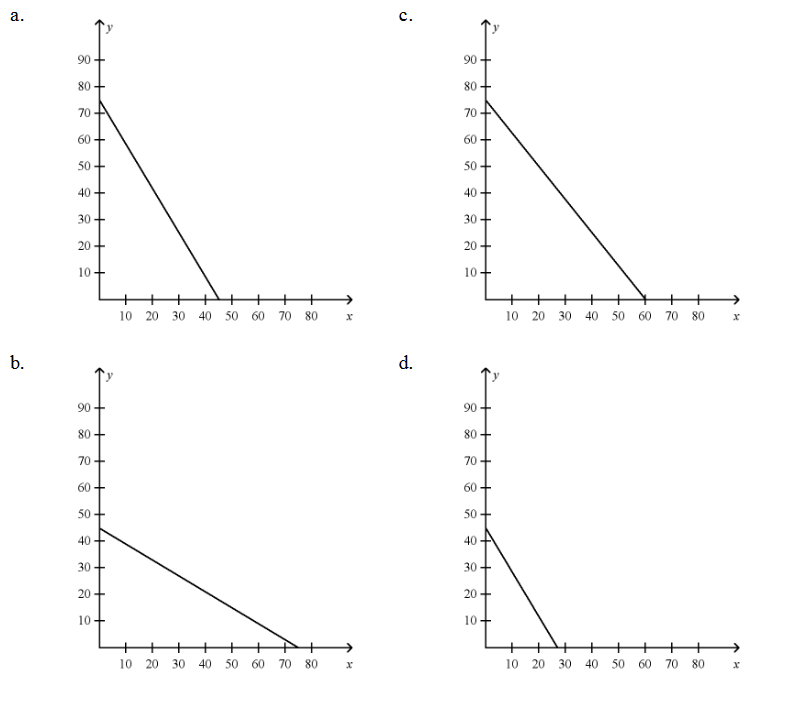11 / 40 If you graph y = x² − 6x + 9, the y-intercept of the graph of the equation is ___________. 12 / 40 For f(x) = 24 − 2x, find f(2) and find x such that f(x) = 10 13 / 40 Multiply: (a + b)(a − b) 14 / 40 Factor p² − 40 15 / 40 The diagram shows a Venn diagram for sets A and B. What is the intersection? Set A: factors of 9 Set B: factors of 1216 / 40 The height of a ball in feet is modeled by y = −16x2 + 72x, where x is the time in seconds after the ball is hit. How long is the ball in the air?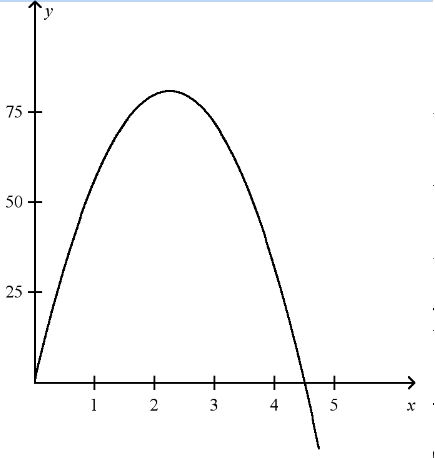17 / 40 Which expression is NOT equivalent to the other expressions? 16x 4 y 2 18 / 40 Which of the following is the equation of the line that has x-intercept = −2 and y-intercept = −4 ? 19 / 40 Subtract (6a2 + 3a) − (4a2 + 2a). 20 / 40 Leah scored p points in the first half of the basketball game. In the second half, she scored 3 more than ½ the number of points she scored in the first half of the game. Altogether, she scored 21 points in the game. The following equation represents this situation where p represents the number of points Leah scored in the first half.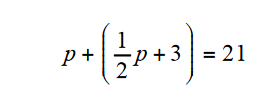How many points did Leah score in the first half? 21 / 40 Let A = {a, b, d, f, g} and B be a sets in the universe U = {letters of the alphabet}. If AB = {b, d}, which could be set B? 22 / 40 Tell whether the slope of the line is positive, negative, zero, or undefined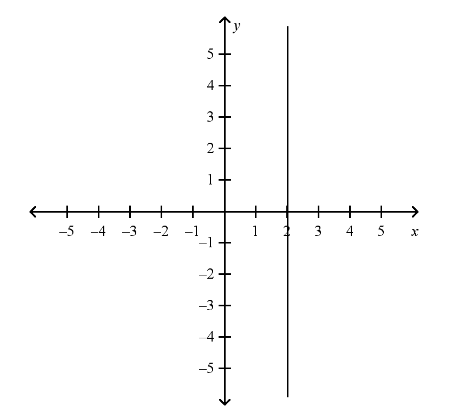23 / 40 Gloria earns 1.5 times her normal hourly pay for each hour that she works over 40 hours in a week. Her normal pay is p dollars per hour. Last week Gloria worked 47 hours and earned$489.85. The following equation represents this situation where p is Gloria’s normal hourly pay in dollars per hour.

40p + 7(1.5p) = 489.85

What is Gloria’s normal hourly pay?

24 / 40

Given f(x) = x 2 + 1 with domain D: {−2, −1, 0, 1, 3 } . What is the range, R?

25 / 40

A sales clerk earns a 3% commission on each sale. What is the commission earned on a sale of \$4450?

26 / 40

Look at the map below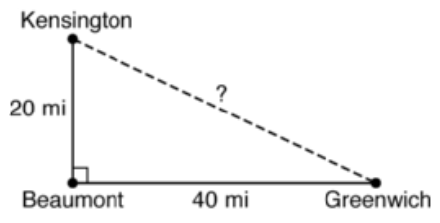Which is the distance between Kensington and Greenwich?

27 / 40

30 people were asked if they wore a blue shirt or a red shirt this week. The Venn diagram shows the results of the survey.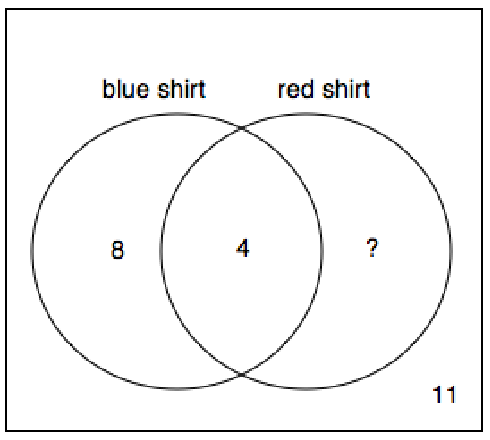What is the missing value in the Venn diagram?

28 / 40

Which system has no solution?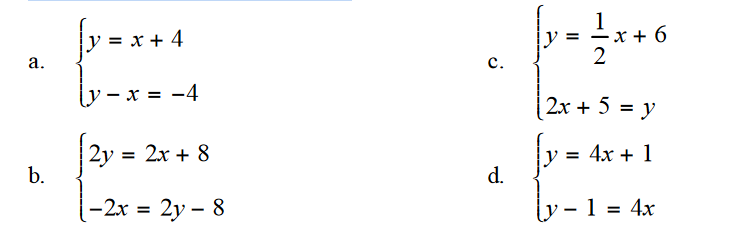29 / 40

Simplify the expression \sqrt[]{\frac{48}{147}}

30 / 40

Simplify (a3b)2.

31 / 40

Which of the following relations is a function?

32 / 40

Graph the line with the slope ½ and y-intercept 3.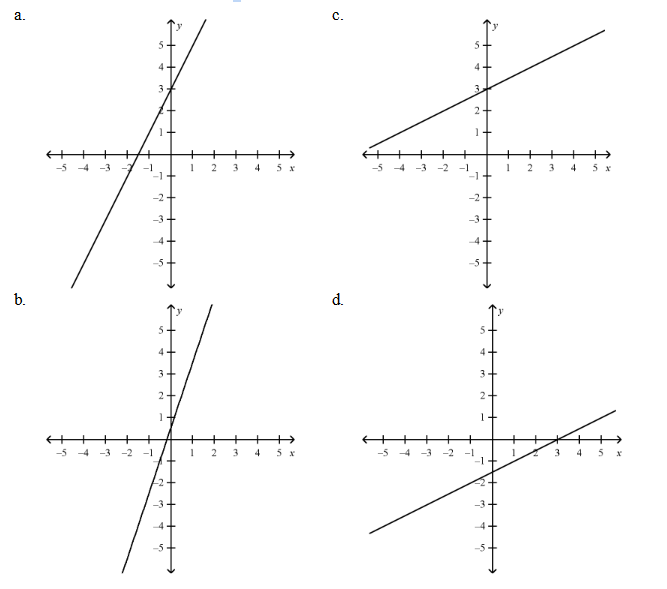33 / 40

Solve m − 8 ≤ 14.

34 / 40

What is the equation of the line shown in the graph?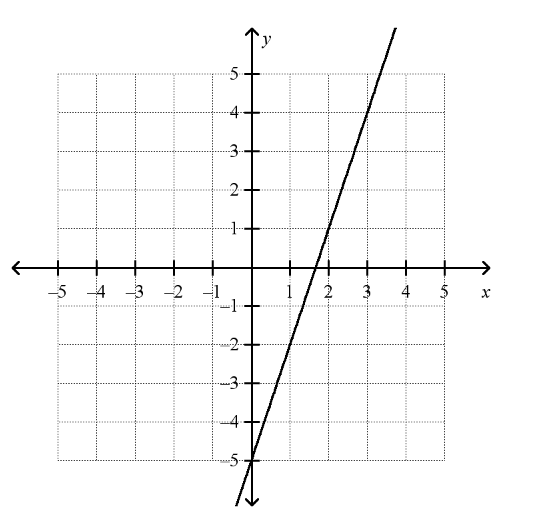35 / 40

The average of Paula’s two test scores must be 80 or more for her to get at least a B in the class. She got a 72 on her first test. What grades can she get on the second test to make at least a B in the class?

36 / 40

The ratio of boys to girls in a class is 2:3. If there are 18 girls in the class, how many boys are there?

37 / 40

U is the set of natural numbers less than 8. G is the set of even integers less than 10. Which is the complement of set G in universe U?

38 / 40

Which of the following is the solution to this inequality?

3(5 + 2n) ≥ 7 + 10n

39 / 40

Divide: (18x3 + 9x2) ÷ (3x)

40 / 40

Use the zero product property to solve the equation x + 3( ) x − 2( ) = 14.